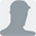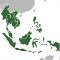Path: EDN Asia >> Design Centre >> Computing/Peripherals >> Grasping the propagating plane wave

# Grasping the propagating plane wave

05 May 2015  | Roy McCammon

If you have studied electromagnetic (EM) field theory, you may know that somehow the electric and magnetic fields in a propagating EM wave generate each other to create a self-sustaining wave. This can be proven using vector algebra, partial derivatives and curl. Few people who get that far in their studies can follow the math. Among those can follow the math, few understand how it works.

This article will show with pictures how the fields of a plane wave interact to generate each other with almost no math. To do this, we will introduce two ideas that may be unfamiliar to some readers. The first idea is that ∂D/∂t and ∂B/∂t (the underappreciated fields) are legitimate fields and should be treated as such. In particular, it is useful to depict these fields along with E, H, B and D.

The second idea is that a current composed of magnetic monopoles would be associated with the E field in the same way that a current of electrons is associated with the H field.

You already know that sending current through a wire coiled into a solenoid shape can create a strong magnetic field inside the solenoid. We will build on that knowledge. But before we do that, we need to define some symbols.

Definitions:

E Electric Intensity

D Electric Flux Density or Electric Displacement Density

H Magnetic Intensity

B Magnetic Flux Density or Magnetic Displacement Density

D/∂t Electric Displacement Current Density

B/∂t Magnetic Displacement Current Density

The word cause (and related words such as create or generate) will be used in a loose manner. For instance, if we were talking about ohms law, V = I∙R, it might be convenient to say that the current causes the voltage or it might be convenient to say that the voltage causes the current. Given R, current can be derived from voltage or voltage can be derived from current. We will say X causes Y or X creates Y because that is the language we normally use, but we will mean that X and Y have a relationship such that complete knowledge of X mathematically implies complete knowledge of Y and that the result would be the same if X were the actual cause of Y.

We also need to lightly cover a few unfamiliar topics.

Magnetic Current: Ostensibly, a current composed of moving magnetic monopoles. Just as electric monopoles (electrons for example) in motion constitute an electric current, magnetic monopoles (if they existed) in motion would constitute a magnetic current. There are no magnetic monopoles, but if there were, Maxwell's equations are readily extensible to include magnetic charge and magnetic currents. Sometimes, when analyzing antennas or other EM field problems, the problem can be made easier by transforming the problem to its dual. This can give rise to non-physical magnetic currents. Harrington¹ discusses the generalized Maxwell's equations and Balanis² discusses how magnetic currents are sometimes used to analyze antennas. Just as electric currents are accompanied by a magnetic (H) field, magnetic currents are accompanied by an electric (E) field. Magnetic current following a solenoid path would create a strong E field inside the solenoid analogously to an electric current following a solenoid path creating a strong H field inside the solenoid. All you need to know about magnetic currents is that they are just like ordinary electric currents except they are accompanied by an E field instead of an H field.

Displacement Current Densities: You may know that ∂D/∂t is called the electric displacement current density. You may have never heard of a name for ∂B/∂t but you probably would not be surprised to know that it is called the magnetic displacement current density. The value of ∂D/∂t field at a particular point in space and time is the partial derivative of D with respect to time at that same point in space and time. ∂B/∂t has a similar relation to B. According to textbooks and from where they occur in Maxwell's equations, we know that ∂D/∂t and ∂B/∂t act exactly like an electric and magnetic current densities respectively. A classical vacuum is empty, so it may seem paradoxical that there could be a current in the vacuum. It may seem doubly paradoxical in the case of magnetic displacement current. Not only is there a current in a supposedly empty vacuum, but it acts like it is made on non-existent magnetic monopoles. In classical physics, there is no satisfactory physical explanation as to the composition of these fields in a vacuum. We will take the pragmatic approach and simply say that if the D and B fields exist, then the ∂D/∂t and ∂B/∂t fields also exist and can be illustrated in the same manner as any other field. All you need to know about ∂D/∂t and ∂B/∂t is to consider them to be ordinary electric and magnetic current densities respectively.Figure 1: Displacement currents as sources of E and H.

Want to more of this to be delivered to you for FREE?

Subscribe to EDN Asia alerts and receive the latest design ideas and product news in your inbox.

Got to make sure you're not a robot. Please enter the code displayed on the right.Time to activate your subscription - it's easy!

We have sent an activate request to your registerd e-email. Simply click on the link to activate your subscription.*Verify code:Control this smart glass with the blink of an eye